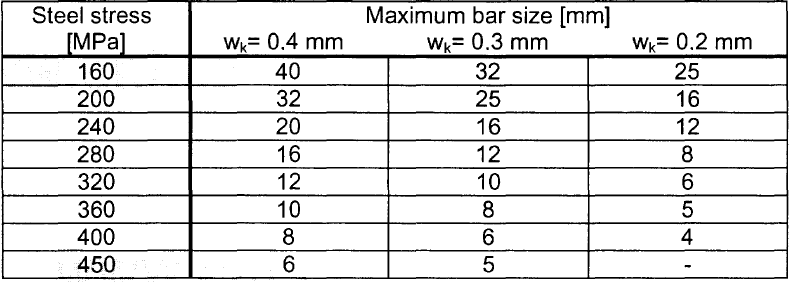# RF-CONCRETE Members – Online Manual Version 5

Online manuals, introductory examples, tutorials, and other documentation.

# 2.2.3 Minimum Reinforcement

### Minimum Reinforcement

The minimum reinforcement area for crack control is determined according to EN 1992-1-1, clause  7.3.2 (2), Eq. (7.1), simplified as follows:

Equation 2.10 EN 1992-1-1, Eq. (7.1)

where

• As,min : minimum area of reinforcing steel in tension zone
• σs : allowable stress of reinforcing steel according to Figure 2.3
• kc : factor for considering stress distribution in tension zone
• kc = 1.0 for pure tension
• kc = 0.4 for bending
• For bending with axial force, kc is determined as follows:

Equation 2.11 EN 1992-1-1, Eq. (7.2)

• where
• σc : mean concrete stress acting on part of section under consideration
σc = NEd / (b ⋅ h)
• NEd : axial force acting on part of cross-section under consideration
• h* = h < 1.0 m
• k1 : coefficient for considering effects of axial forces on stress distribution:
• k1=1.5 for NEd = compressive force
k1 = 2h*/3h for NEd = tensile force
• k : coefficient to consider nonlinearly distributed self-equilibrating stresses
• k = 1.0 for webs with h ≤ 300mm
• k = 0.65 for h ≥ 800 mm
• k = 1.0 for restraint caused outside (e.g. column settlement)
• fct,eff = fctm : mean value of effective tensile strength of concrete when cracks occur
• Act : area of concrete tension zoneFigure 2.3 Limit diameter Øs* for reinforcing steels according to EN 1992-1-1, Table 7.2